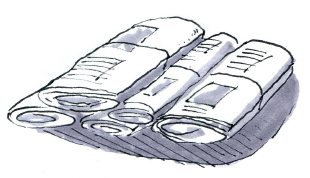### Home > INT1 > Chapter 10 > Lesson 10.1.1 > Problem10-10

10-10.

In a certain small town, $65$% of the residents subscribe to the city Sunday paper, 37% subscribe to the weekly local paper, and 25% subscribe to both papers.1. What are the two variables? Create a two-way table to represent the data.

 Subscribes toweekly localpaper Subscribes to Sunday paper yes no yes $0.25$ $\mathbf{0.37}$ no $\mathbf{0.65}$ $\mathbf{1.00}$
2. If a resident is selected at random, what is the probability he or she subscribes to at least one of the two papers?

Which three boxes in the two-way table represent someone subscribing to at least one paper?

$25\%+12\%+40\%=77\%$

1. Charlie’s neighbor subscribes to a paper. What is the probability that he takes the Sunday paper?

This is the probability of someone subscribing to the Sunday paper given the information that they subscribe to at least one paper.
$\frac{65}{77}=?$